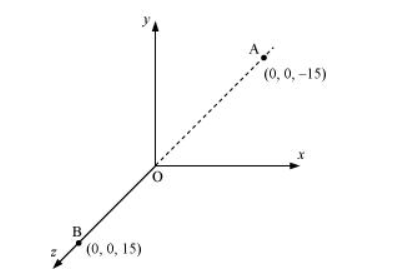# A system has two chargesQuestion:

A system has two charges $q_{A}=2.5 \times 10^{-7} C$ and $q_{B}=-2.5 \times 10^{-7} C$ located at points $A$ : $(0,0,-15 \mathrm{~cm})$ and $B$ : $(0,0,+15 \mathrm{~cm})$, respectively. What are the total charge and electric dipole moment of the system?

Solution:

Both the charges can be located in a coordinate frame of reference as shown in the given figure.At $A$, amount of charge, $q_{A}=2.5 \times 10^{-7} \mathrm{C}$

At $\mathrm{B}$, amount of charge, $q_{\mathrm{B}}=-2.5 \times 10^{-7} \mathrm{C}$

Total charge of the system,

$q=q_{A}+q_{B}$

$=2.5 \times 10^{-7} \mathrm{C}-2.5 \times 10^{-7} \mathrm{C}$

= 0

Distance between two charges at points A and B,

d = 15 + 15 = 30 cm = 0.3 m

Electric dipole moment of the system is given by,

$p=q_{A} \times d=q_{B} \times d$

$=2.5 \times 10^{-7} \times 0.3$

$=7.5 \times 10^{-8} \mathrm{C}$ m along positive $z$-axis

Therefore, the electric dipole moment of the system is $7.5 \times 10^{-8} \mathrm{C} \mathrm{m}$ along positive $z$-axis.